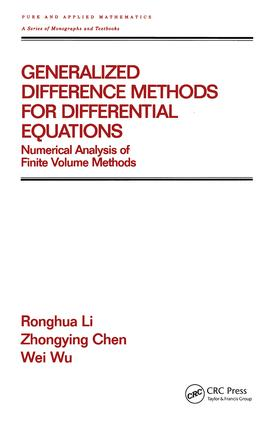# Generalized Difference Methods for Differential Equations

## Numerical Analysis of Finite Volume Methods, 1st Edition

CRC Press

466 pages

##### Purchasing Options:\$ = USD
Hardback: 9780824703301
pub: 2000-01-03
SAVE ~\$60.00
\$300.00
\$240.00
x

FREE Standard Shipping!

### Description

This text presents a comprehensive mathematical theory for elliptic, parabolic, and hyperbolic differential equations. It compares finite element and finite difference methods and illustrates applications of generalized difference methods to elastic bodies, electromagnetic fields, underground water pollution, and coupled sound-heat flows.

### Reviews

". . .an extraordinary text presenting, mathematically, delicate problems of numerical approximations in a very careful and yet accessible manner. "

---Mathematical Reviews

"…contains a lot of very useful material on the numerical analysis of the finite volume scheme…. …useful to researchers and graduate students working on the numerical analysis of finite element methods and finite volume methods for elliptic problems."

---Zentralblatt fur Mathematik, 2000

"…an indispensable reference work for any numerical analyst who studies and uses finite difference, finite element, and finite volume methods and other approaches for solving partial differential equations and applications. Besides its value as an excellent reference book for the generalized difference methods or for some finite volume methods from the point of view of the generalized finite element method, many ideas, both in algorithm design and theoretical analysis, can be applied elsewhere."

---SIAM Review, 2001

Preliminaries; two point boundary value problems; second order elliptic equations; fourth order and nonlinear elliptic equations; parabolic equations; hyperbolic equations; convection-dominated diffusion problems; applications.

### Subject Categories

##### BISAC Subject Codes/Headings:
MAT000000
MATHEMATICS / General
MAT021000
MATHEMATICS / Number Systems